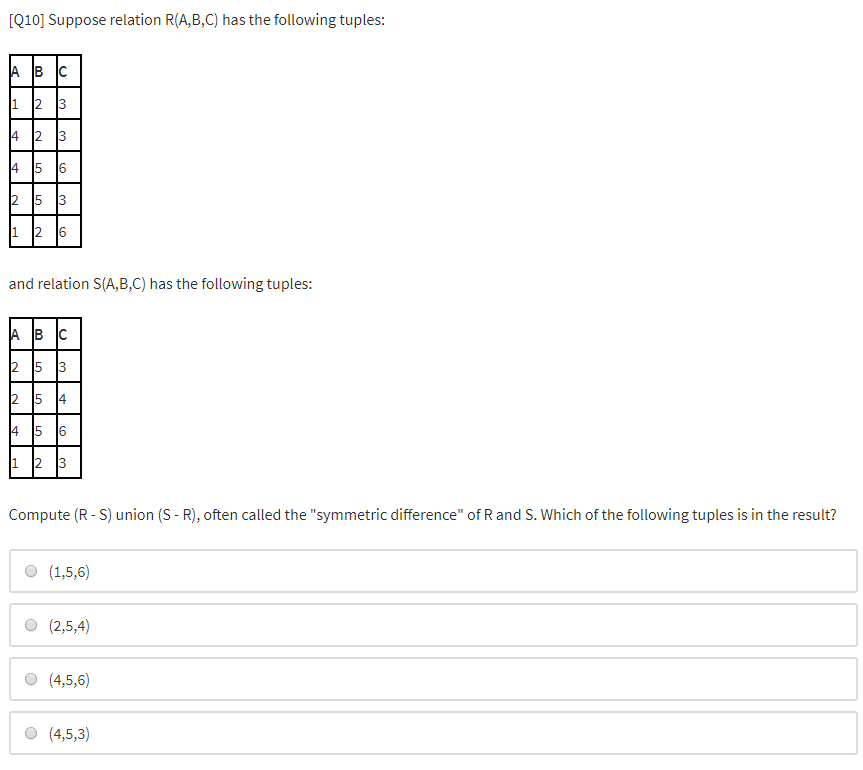### Create an Account

Home / Questions / [Q10] Suppose relation R(A,B,C) has the following tuples: ? ? ? 1 2 4 4 6 1 2 6 and relation S(A, B,

# [Q10] Suppose relation R(A,B,C) has the following tuples: ? ? ? 1 2 4 4 6 1 2 6 and relation S(A, B,[Q10] Suppose relation R(A,B,C) has the following tuples: А В С 1 2 4 4 6 1 2 6 and relation S(A, B,C) has the following tuples: А В с 2 5 3 2 5 4 4 6 1 2 3 Compute (R - S) union (S- R), often called the "symmetric difference" of R and S. Which of the following tuples is in the result? (1,5,6) (2,5,4) (4,5,6) (4,5,3) LO LO LO

Apr 02 2020 View more View LessSubscribe To Get Solution